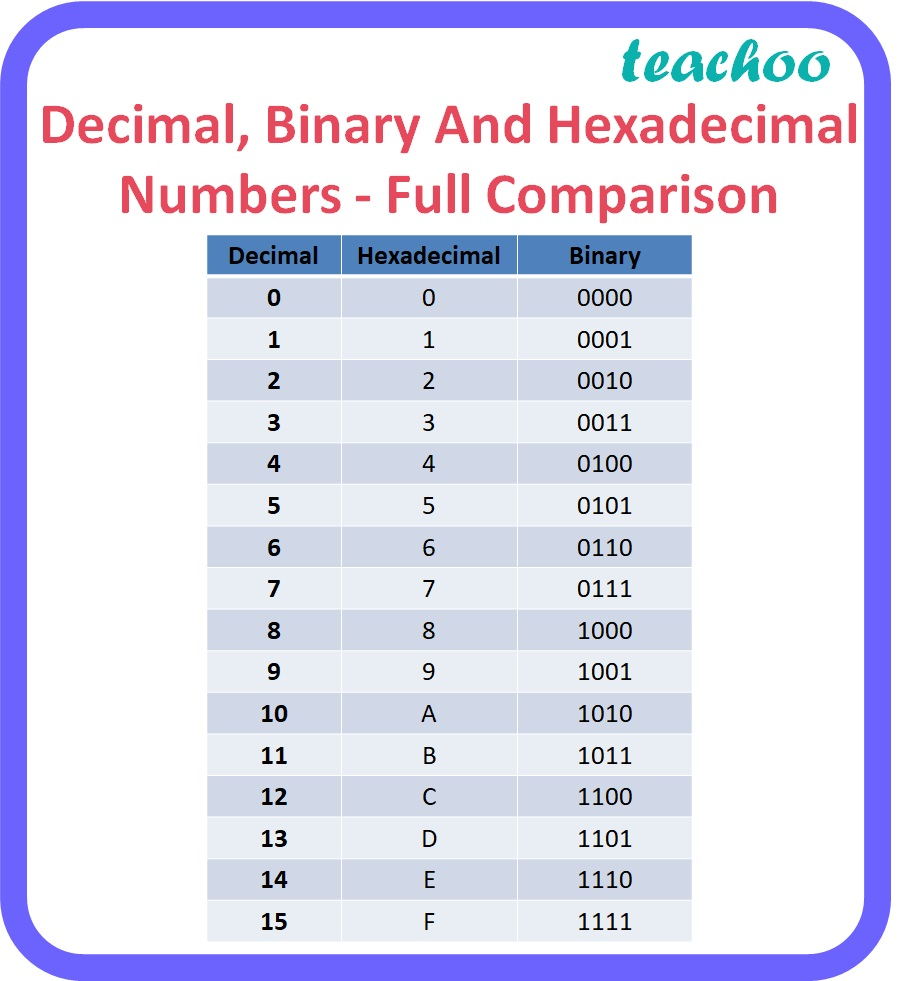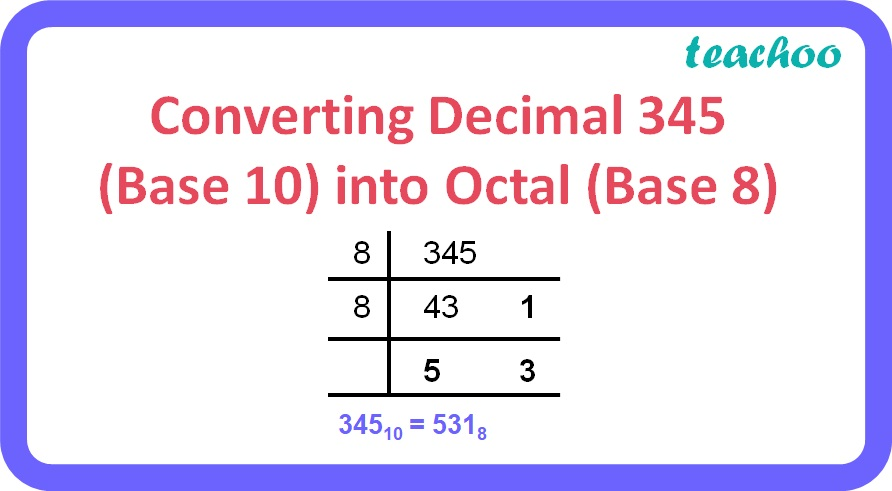5 Mark Questions

Computer Science - Class 11
Chapter 2 Class 11 - Encoding Schemes and Number System

## (c) Convert the Decimal number 345 into Octal number

### (a) 3BC 16 to its binary equivalent

 3 B C 0011 1011 1100

Therefore, 3BC 16 = 001110111100 2

Explanation:

• To obtain the binary equivalent of a hexadecimal number, individual digits of the hexadecimal number should be converted to binary in groups of four digits as given in the table below.### (b) 10011010.010101 2 to its hexadecimal equivalent

 1001 1010 0101 01 00 9 A 5 4

Therefore, 10011010.010101 2 = 9A.54 16

Explanation:

• To obtain the Hexadecimal equivalent of a binary number, digits of binary number should be divided into groups of four digits starting from the rightmost digit for the integer part and starting from the leftmost digit for the fractional part.
• Convert each group of 4 digits to one hexadecimal digit as given in the table below.
• Note: 1,2 or 3 zeroes can be added before the leftmost digit or after the rightmost digit to make a complete group of 4 bits.### (c) 345 10 to its octal equivalentTherefore, 345 10 = 531 8

Explanation:

• Repeatedly divide the decimal number by 8 until the quotient becomes less than 8 and record all the remainders.
• The remainders should be written bottom to upwards to get the octal equivalent of the decimal number.

Learn in your speed, with individual attention - Teachoo Maths 1-on-1 Class